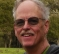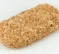## Curve ModellingStarted by 4 years ago7 replieslatest reply 4 years ago181 views

Hi All,

I hope this question is OK here! If not, I'll go away...quickly.

I  want to understand how an estimation for a curve fit polynomial could be created. I'm not asking for one to be made...just how to go about it.

I have a lithium battery, and the data sheet has various curves of temperature for discharge rate of current (x-axis) against voltage (y-axis) . I imagine one way to do it is to create a table and interpolate between those points. It's rudimentary and would be close...but, by the curves, may not be close enough. Unless I enter a lot of points! I can't afford a voltage bump pulling too much current and would be better if I could get it as close as possible.

It would be ideal to pass in the x axis desired current at a specific temperature and see the resultant voltage. I imagine each temperature curve would have it's own cure fit polynomial. So what is the right way to go about this?

Thanks!

[ - ]Hi Groger, not trying to be unkind here but did you try typing "how to estimate a polynomial curve fit" into box at www.google.com ? If so it would have shown you this link:

www.math.tamu.edu › ~glahodny › Math442 › Curve Fitting

### Curve Fitting and Parameter Estimation - TAMU Math

curve that best fits this data. The most common form of curve fitting is linear least squares regression. 1.1 Polynomial Regression. In order to develop an idea of ...

Which is a wonderful discussion of the techniques using MATLAB. I you don't have a MATLAB license (you can get a demo license for free) you can run gnu OCTAVE which has the same syntax and does the same stuff for free. There are versions for Windows, Linux and MacOS.

My goal here is to get your toolkit buffed with some of the tools you will find useful in day to day explorations of this space! If videos are more your style, watching the curve fitting videos on YouTube from Khan Academy () are also really great and full of all the bits you need with exercises to test your understanding. I can easily recommend them.

[ - ]If you use Matlab, then I think these 2 very simple lines of code sum up Least Squares polynomial curve fitting very well:

C = bsxfun(@power, (0:L).', (0:N));
a = pinv(C)*y;

(where 0:L are the sample time indices, and N is the desired polynomial order). The first line simply creates a (L+1 x N+1) matrix, C, whose (n,p)th element is np. The second line forms the Moore-Penrose pseudoinverse of C and multiplies this with the observed data vector, y. The vector a then contains the coefficients of the Least Squares best fit polynomial.

I wrote some notes about this in a blog here but sadly the equation rendering is now broken on that site. I could move it into a blog here at dsprelated if it's of any interest.

[ - ]Hello Groger, I am not sure to fully appreciate your problem and probably the previous answers would do the job nicely, but what strikes me is that you have a family of fits not only just one. If you want a parcimonious solution may be you should consider a 2-dimensionnal fit. You may consider your family of curves current I versus voltage V indexed by temperature T as the 2 dimensional cuts of a 3D function T(I,V). The interpolation problem may require base functions that differ from polynomials but if they are well chosen they may be very few. I am speculating... the only way to be sure is to look at your data.

Regards

[ - ]I usually try 1st with Wolfram|Alpha
[ - ]Hi,

Thanks for all of your answers! Appreciated. This is happening in a microcontroller so I have to derive the equation first and then apply the x-variable (current) for a specific T. The T isn't an issue, since I read that accurately.

I found this method on a youtube video and it seems that it will work good, since I have a set of given points and need only apply the x-variable of interest. It's called "Determine Polynomial equation from given set of data points by Finite Difference"

[ - ]If I understand what you are trying to do, then I don't think the method of finite difference is the best choice. As far as I know, the method of finite difference allows you to find the coefficients of an exact polynomial.

Of course, if you allow the order of the polynomial to be very large (as large as your number of data points), then you'll eventually be able to find an exact polynomial expression for any practical sequence of values.

However, I think what we usually want is some relatively simple (low order) polynomial expression that closely models the underlying system (and not the random effects of measurement errors and noise). This is what the 2 lines of Maltab code in my previous post do.

[ - ]